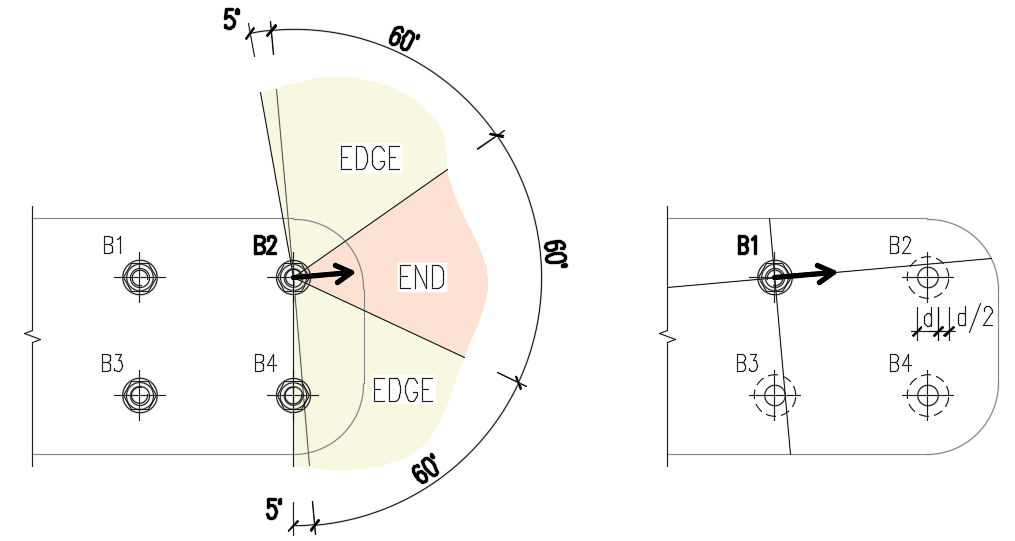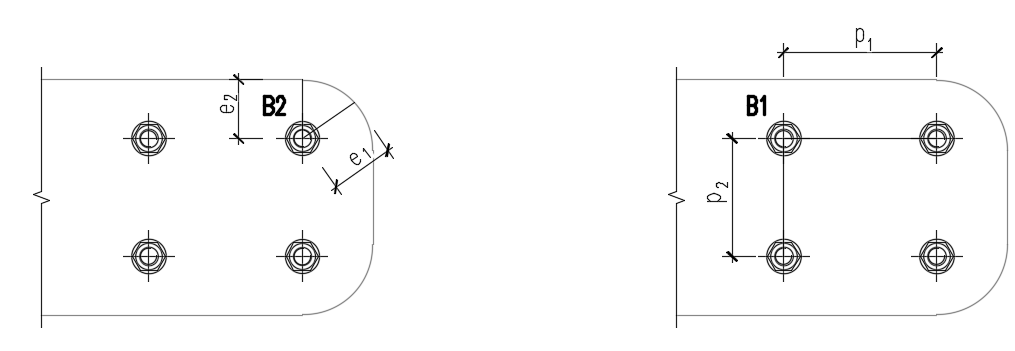### Choose language# Bolt bearing distances for Eurocode

$$The new version brings an improved algorithm for calculating the bolt spacing (p1; p2), end (e1), and edge (e2) distances for the Eurocode bearing check. This improvement is mostly relevant for general plate geometries, plates with openings, cutouts, etc.The algorithm reads the real direction of the resulting shear force vector in a given bolt and then calculates the distances needed for the bearing check.The end (e1) and edge (e2) distances are determined by dividing the plate contour into three segments. The end segment is indicated by a 60° range in the direction of the force vector. The edge segments are defined by two 65° ranges perpendicular to the force vector. The shortest distance from a bolt to a relevant segment is then taken as an end, or an edge distance.The spacing distances between bolt holes (p1; p2) are determined by virtually enlarging the surrounding bolt holes by a half of their diameter, then drawing two lines in direction and perpendicular to the shear force vector. The distances to the enlarged bolt holes that are intersected by these lines are then considered as p1 and p2 in the calculation.Available in Expert and Enhanced edition.$$